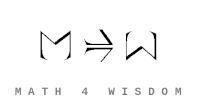文章 发现 ms@ms.lt +370 607 27 665 My work is in the Public Domain for all to share freely. 读物 书 影片 维基百科 Software Upload Understand the Univalence Axiom and Voevodsky's homotopy type theory in terms of my philosophical concepts. 同伦类型论 . . . Homotopy type theory Relate the univalence axiom {$(A=B) \simeq (A\simeq B)$} and the Yoneda lemma {$F(A)\cong\{\{A\rightarrow\_\}\rightarrow F(\_)\}$}. Study the kinds of equivalences. Associate them to the relationship between level and metalevel. Relate spectral sequences, exact sequences and divisions of everything. Understand what homotopy type theory has to say about the homotopy groups of spheres and thus Bott periodicity. What is the relationship between loops - identity paths in Homotopy Type Theory - and strings in string theory? Readings Videos Community Vilniaus universitetas. Linas Laibinis domisi Voevodskiu ir t.t. Rimvydas Krasauskas's interests New foundations for mathematics Having a real programming language to check proofs Concepts: Different levels of equivalences Paths between points (homotopies) and equivalence of homotopies Homotopy Type Theory Lecture Notes In shulman see discussion of space and adjoint string and local topos. In shulman how are dependent sum and dependent product adjoints?
Šis puslapis paskutinį kartą keistas November 05, 2021, at 01:27 PM